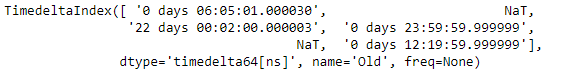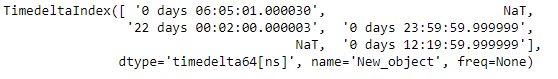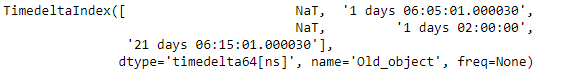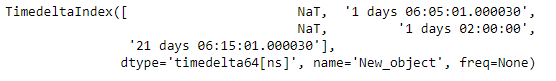Open in App
Not now

# Python | Pandas TimedeltaIndex.rename

• Last Updated : 06 Jan, 2019

Python is a great language for doing data analysis, primarily because of the fantastic ecosystem of data-centric python packages. Pandas is one of those packages and makes importing and analyzing data much easier.

Pandas` TimedeltaIndex.rename()` function set new names on index. It defaults to returning new index.

Syntax : TimedeltaIndex.rename(name, inplace=False)

Parameters : None
name : [str or list] name to set
inplace : if True, mutates in place

Return : new index (of same type and class…etc) [if inplace, returns None]

Example #1: Use `TimedeltaIndex.rename()` function to rename the given TimedeltaIndex object.

 `# importing pandas as pd``import` `pandas as pd`` ` `# Create the TimedeltaIndex object``tidx ``=` `pd.TimedeltaIndex(data ``=``[``'06:05:01.000030'``, ``None``, ``'22 day 2 min 3us 10ns'``,``                      ``'+23:59:59.999999'``, ``None``, ``'+12:19:59.999999'``], name ``=``'Old'``)`` ` `# Print the TimedeltaIndex object``print``(tidx)`

Output :Now we will use the `TimedeltaIndex.rename()` function to rename the tidx object.

 `# rename the tidx object``tidx.rename(name ``=``'New_object'``)`

Output :As we can see in the output, the `TimedeltaIndex.rename()` function has returned a new index and has set the name attribute of the TimedeltaIndex object.

Example #2: Use `TimedeltaIndex.rename()` function to rename the given TimedeltaIndex object.

 `# importing pandas as pd``import` `pandas as pd`` ` `# Create the TimedeltaIndex object``tidx ``=` `pd.TimedeltaIndex(data ``=``[``None``, ``'1 days 06:05:01.000030'``, ``None``, ``'1 days 02:00:00'``,``                                         ``'21 days 06:15:01.000030'``], name ``=``'Old_object'``)`` ` `# Print the TimedeltaIndex object``print``(tidx)`

Output :Now we will use the `TimedeltaIndex.rename()` function to rename the tidx object.

 `# rename the tidx object``tidx.rename(name ``=``'New_object'``)`

Output :As we can see in the output, the `TimedeltaIndex.rename()` function has returned a new index and has set the name attribute of the TimedeltaIndex object.

My Personal Notes arrow_drop_up